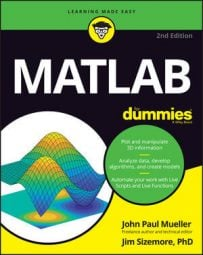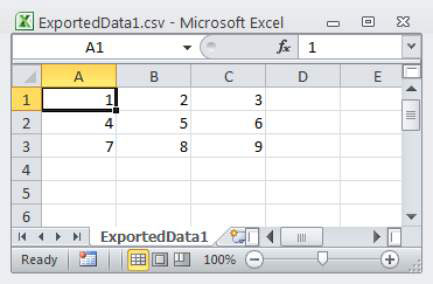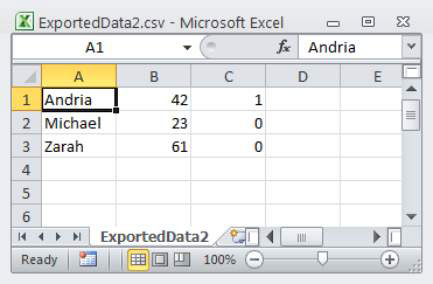##### MATLAB For DummiesWhen you have data inside MATLAB and want to export it, the goal is to ensure that the resulting file is standardized so that the recipient has minimum problems using it. As a result, you’d use writetable() only if the recipient really did require a custom format rather than a standard .csv file, or if the MATLAB data was such that you had to use something other than csvwrite().

## Working with matrices and numeric data

Before you can do anything with exporting, you need data to export. Type ExportMe = [1, 2, 3; 4, 5, 6; 7. 8, 9] and press Enter. You see the following result:

```ExportMe =
1  2  3
4  5  6
7  8  9```

The result is a matrix of three rows and three columns. Exporting matrices is simple because the majority of the functions accept a matrix as a default. To see how exporting matrices works, type csvwrite(‘ExportedData1.csv’, ExportMe) and press Enter. MATLAB creates the new file, and you see it appear in the Current Folder window. (What you see precisely will vary depending on the application you use to view .csv files.)Not all MATLAB data comes in a convenient matrix. When you use csvwrite(), you must supply a matrix. To get a matrix, you may have to convert the data from the existing format to a matrix using a conversion function. For example, when the data appears as a cell array, you can use the cell2mat() function to convert it.

However, some conversions aren’t so straightforward. For example, when you have a table as input, you need to perform a two-step process:

1. Use the table2cell() function to turn the table into a cell array.

2. Use the cell2mat() function to turn the cell array into a matrix.

## Working with mixed data

Exporting simple numeric data is straightforward because you have a number of functions to choose from that create the correct formats directly. The problem comes when you have a cell array or other data form that doesn’t precisely match the expected input for csvwrite(). To see how mixed data works, start by typing MyCellArray = {‘Andria’, 42, true; ‘Michael’, 23, false; ‘Zarah’, 61, false} and pressing Enter.

You see the following result:

```MyCellArray =
‘Andria’   
‘Michael’  
‘Zarah’   ```

The mixed data type is a problem. If the data were all one type, you could use the cell2mat() function to convert the cell array to a matrix like this: MyMatrix = cell2mat(MyCellArray). Unfortunately, if you try that route with the data in MyCellArray, you see the following error message:

```Error using cell2mat (line 46)
All contents of the input cell array must be of the same data type.```

To obtain the required .csv file output, you must first convert the cell array into something else. The easiest approach is to rely on a table. Type MyTable = cell2table(MyCellArray) and press Enter. You obtain the following output:

```MyTable =
MyCellArray1 MyCellArray2 MyCellArray3
____________ ____________ ____________
‘Andria’  42    true
‘Michael’  23    false
‘Zarah’   61    false ```

At this point, you can type writetable(MyTable, ‘ExportedData2.csv’, ‘WriteVariableNames’, false) and press Enter. The output will use commas as delimiters between columns, so most applications will see the resulting file as true .csv format. This is what the output looks like in Excel. (Your output may vary based on the application you use to view it.)In this case, the exporting process works fine. However, you can always use properties to fine-tune the output of writetable(), just as you do with readtable(). Here is a quick overview of the writetable() properties and their uses:

• FileType: Defines the type of file. The two acceptable values are text and spreadsheet.

• WriteVariableNames: Specifies whether the first row of the output file contains variable names used in MATLAB. The acceptable values are true (default), false, 1, or 0.

• WriteRowNames: Specifies whether the first column of the output file contains the row names used in MATLAB. The acceptable values are true, false (default), 1, or 0.

• Delimiter: Defines which characters are used as delimiters. You specify this value as a string of individual delimiter characters.

• Sheet: Indicates which worksheet to write in the file. The acceptable values are 1 (default), any positive integer indicating the worksheet index, or a string containing the worksheet name. The worksheets are written one at a time, so you need multiple calls to write multiple worksheets.

• Range: Specifies the rectangular portion of worksheet to write. When the MATLAB data exceeds the size of the range, the data is truncated and only the data that will fit appears in the output file. This value is supplied as a string.